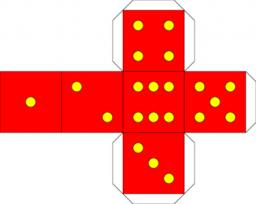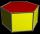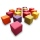# The cube

The surface of the cube is 150 square centimeters. Calculate:
a- the content of its walls
b - the length of its edges
c - its volume

S1 =  25 cm2
a =  5 cm
V =  125 cm3

### Step-by-step explanation:Did you find an error or inaccuracy? Feel free to write us. Thank you!Tips to related online calculators
Tip: Our volume units converter will help you with the conversion of volume units.

#### You need to know the following knowledge to solve this word math problem:

We encourage you to watch this tutorial video on this math problem:

## Related math problems and questions:

• Cube 5The content area of one cube wall is 32 square centimeters. Determine the length of its edges, its surface and volume.
• The cubeThe cube has a surface area of 486 m ^ 2. Calculate its volume.
• Cube surfce2volumeCalculate the volume of the cube if its surface is 150 cm2.
• The cubeThe cube has a surface of 600 cm2. What is its volume?
• Cuboid - VabFind the surface of the cuboid when its volume is 52.8 cubic centimeters, and the length of its two edges is 2 centimeters and 6 centimeters.
• The cubeThe cube has a surface area of 216 dm2. Calculate: a) the content of one wall, b) edge length, c) cube volume.
• CubeThe sum of lengths of cube edges is 57 cm. What is its surface and volume?
• TerezaThe cube has an area of base 256 mm2. Calculate the edge length, volume, and area of its surface.
• The rodThe rod has the shape of a regular hexagonal prism with a volume of 32.4 cubic decimetres. What is the content of the base if it is 350 centimeters long? Round to ones.
• Cube V2SThe volume of the cube is 27 dm cubic. Calculate the surface of the cube.
• Area to volumeIf the surface area of a cube is 486, find its volume.
• Cube edgeDetermine the edges of the cube when the surface is equal to 37.5 cm square.
• Two wallsCalculate the surface area of a cube in m2 if you know that the area of its two walls is 72 dm2.
• Cube wallsFind the volume and the surface area of the cube if the area of one of its walls is 40 cm2.
• Cube edgesThe sum of the lengths of the cube edges is 42 cm. Calculate the surface of the cube.
• Cube and sphereCube with the surface area 150 cm2 is described sphere. What is sphere surface?
• Cuboid to cubeA cuboid with dimensions of 9 cm, 6 cm, and 4 cm has the same volume as a cube. Calculate the surface of this cube.